# New paper: A simple weighted displacement difference test to evaluate place based crime interventions

At the ECCA conference this past spring Jerry Ratcliffe asked if I could apply some of my prior work on evaluating changes in crime patterns over time to make a set of confidence intervals for the weighted displacement quotient statistic (WDQ). The answer to that is no, you can’t, but in its stead I created another statistic in which you can do that, the weighted displacement difference (WDD). The work is published in the open access journal Crime Science.

The main idea is we wanted a simple statistic folks can use to evaluate place based interventions to reduce crime. All you need is pre and post crime counts for you treated and control areas of interest. Here is an excel spreadsheet to calculate the statistic, and below is a screen shot. You just need to fill in the pre and post counts for the treated and control locations and the spreadsheet will spit out the statistic, along with a p-value and a 95% confidence interval of the number of crimes reduced.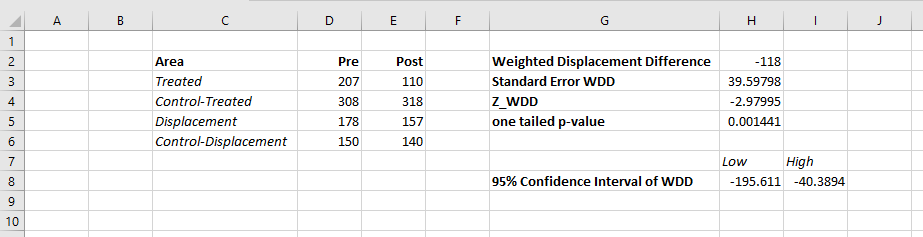What is different compared to the WDQ statistic is that you need a control area for the displacement area too in this statistic. But if you are not worry about displacement, you can actually just put in zero’s for the displacement area and still do the statistic for the local (and its control area). In this way you can actually do two estimates, one for the local effects and one for the displacement. Just put in zero’s for the other values.

While you don’t really need to read the paper to be able to use the statistic, we do have some discussion on choosing control areas. In general the control areas should have similar counts of crime, you shouldn’t have a treatment area that has 100 crimes and a control area that only has 10 crimes. We also have this graph, which is basically a way to conduct a simple power analysis — the idea that “could you reasonably detect whether the intervention reduced crime” before you actually conduct the analysis.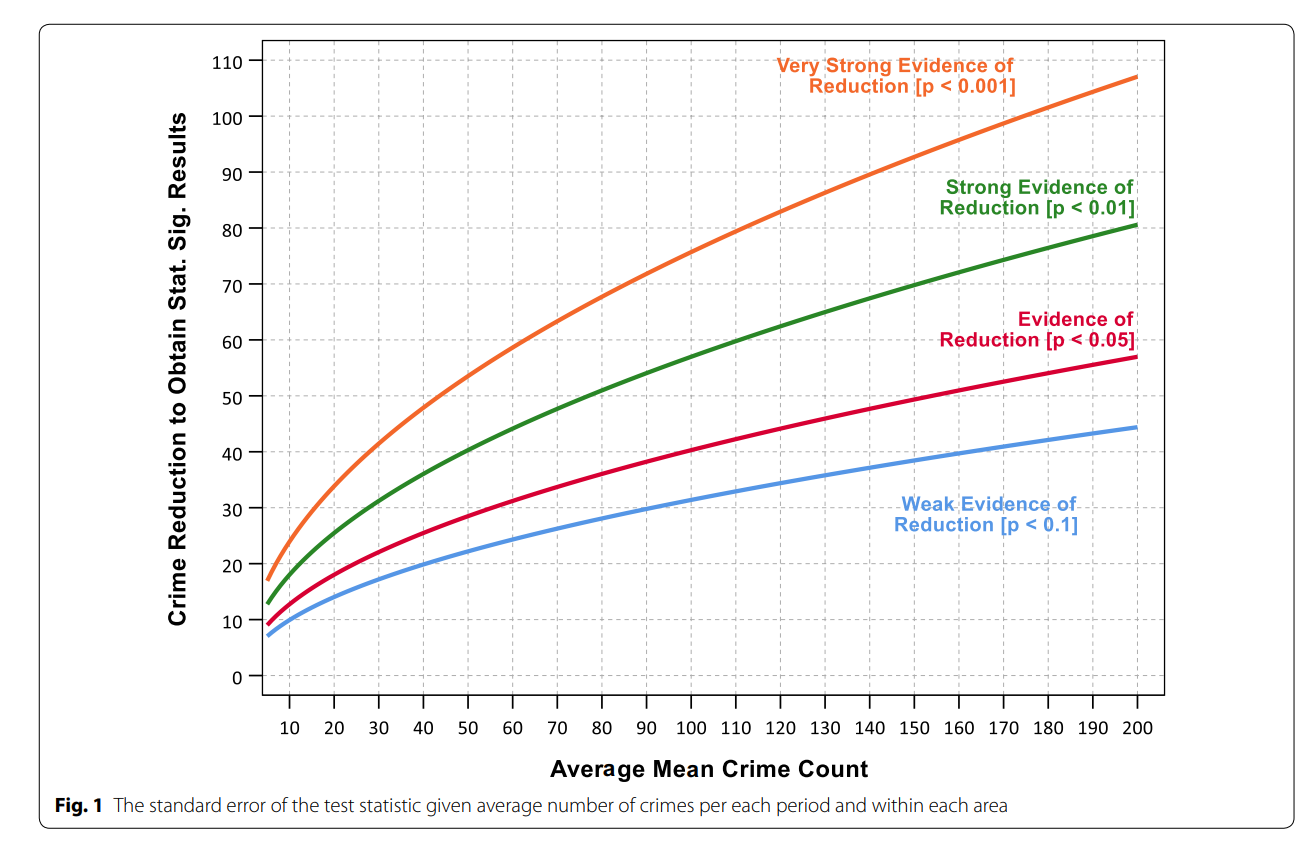So the way to read this graph is if you have a set of treated and control areas that have an average of 100 crimes in each period (so the cumulative total crimes is around 800), the number of crimes you need to reduce due to the intervention to even have weak evidence of a crime reduction (a one-tailed p-value of less than 0.1), the intervention needs to have prevented around 30 crimes. Many interventions just aren’t set up to have strong evidence of crime reductions. For example if you have a baseline of 20 crimes, you need to prevent 15 of them to find weak evidence of effectiveness. Interventions in areas with fewer baseline crimes basically cannot be verified they are effective using this simple of a design.

For those more mathy, I created a test statistic based on the differences in the changes of the counts over time by making an assumption that the counts are Poisson distributed. This is then basically just a combination of two difference-in-difference estimates (for the local and the displacement areas) using counts instead of means. For researchers with the technical capabilities, it probably makes more sense to use a data based approach to identify control areas (such as the synthetic control method or propensity score matching). This is of course assuming an actual randomized experiment is not feasible. But this is too much a burden for many crime analysts, so if you can construct a reasonable control area by hand you can use this statistic.

# Testing changes in short run crime patterns: The Poisson e-test

A common task for a crime analyst is to see if a current set of crime numbers is significantly rising. For a typical example, in prior data there are on average 16 robberies per month, so are the 25 robberies that occurred this month a significant change from the historical pattern? Before I go any further:

PERCENT CHANGE IS A HORRIBLE METRIC — PLEASE DO NOT USE PERCENT CHANGE ANYMORE

But I cannot just say don’t use X — I need to offer alternatives. The simplest is to just report the change in the absolute number of crimes and let people judge for themselves whether they think the increase is noteworthy. So you could say in my hypothetical it is an increase of 9 crimes. Not good, but not the end of the world. See also Jerry Ratcliffe’s different take but same general conclusion about year-to-date percent change numbers.

Where this fails for the crime analyst is that you are looking at so many numbers all the time, it is difficult to know where to draw the line to dig deeper into any particular pattern. Time is zero-sum, if you spend time looking into the increase in robberies, you are subtracting time from some other task. If you set your thresholds for when to look into a particular increase too low, you will spend all of your time chasing noise — looking into crime increases that have no underlying cause, but are simply just due to the random happenstance. Hence the need to create some rules about when to look into crime increases that can be applied to many different situations.

For this I have previously written about a Poisson Z-score test to replace percent change. So in our original example, it is a 56% increase in crimes, `(25-16)/16 = 0.5625`. Which seems massive when you put it on a percent change scale, but only amounts to 9 extra crimes. But using my Poisson Z-test, which is simply `2 * [ Square_Root(Current) - Square_Root(Historical) ]` and follows an approximate standard normal distribution, you end up with:

`2*(sqrt(25) - sqrt(16)) = 2*(5 - 4) = 2`

Hearkening back to your original stats class days, you might remember a z-score of plus or minus 2 has about a 0.05 chance in occurring (1 in 20). Since all analysts are monitoring multiple crime patterns over time, I suggest to up-the-ante beyond the usual plus or minus 2 to the more strict plus or minus 3 to sound the alarm, which is closer to a chance occurrence of 1 in 1000. So in this hypothetical case there is weak evidence of a significant increase in robberies.

The other day on the IACA list-serve Isaac Van Patten suggested to use the Poisson C-test via this Evan Miller app. There is actually a better test than that C-test approach, see A more powerful test for comparing two Poisson means, by Ksrishnamoorthy and Thomson (2004), which those authors name as the E-test (PDF link here). So I just examine the E-test here and don’t worry about the C-test.

Although I had wrote code in Python and R to conduct the e-test, I have never really studied it. In this example the e-test would result in a p-value rounded to 0.165, so again not much evidence that the underlying rate of changes in the hypothetical example.

My Poisson Z-score wins in terms of being simple and easy to implement in a spreadsheet, but the Poisson e-test certainly deserves to be studied in reference to my Poisson Z-score. So here I will test the Poisson e-test versus my Poisson Z-score approach using some simulations. To do this I do two different tests. First, I do a test where the underlying Poisson distribution from time period to time period does not change at all, so we can estimate the false positive rate for each technique. The second I introduce actual changes into the underlying crime patterns, so we can see if the test is sensitive enough to actually identify when changes do occur in the underlying crime rate. SPSS and Python code to replicate this simulation can be downloaded from here.

# No Changes and the False Positive Rate

First for the set up, I generate 100,000 pairs of random Poisson distributed numbers. I generate the Poisson means to have values of 5, 10, 15, 20 and 25. Since each of these pairs is always the same, any statistically significant differences are just noise chasing. (I limit to a mean of 25 as the e-test takes a bit longer for higher integers, which is not a big deal for an analyst in practice, but is for a large simulation!)

Based on those simulations, here is a table of the false positive rate given both procedures and different thresholds.1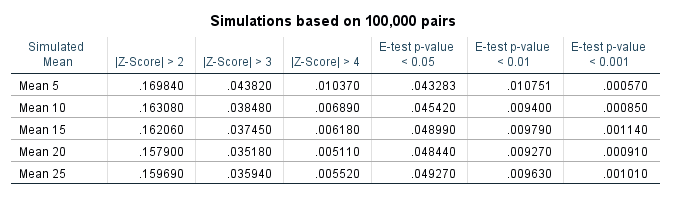So you can see my Poisson Z-score has near constant false positive rate for each of the different means, but the overall rate is higher than you would expect from the theoretical standard normal distribution. My advice to up the threshold to 3 only limits the false positive rate for this data to around 4 in 100, whereas setting the threshold to a Z-score of 4 makes it fewer than 1 in 100. Note these are false positives in either direction, so the false positive rate includes both false alarms for significantly increasing trends as well as significantly decreasing trends.

The e-test is as advertised though, the false positive rate is pretty much exactly as it should be for p-values of less than 0.05, 0.01, and 0.001. So in this round the e-test is a clear winner based on false positives over my Poisson Z-score.

# Testing the power of each procedure

To be able to test the power of the procedure, I add in actual differences to the underlying Poisson distributed random values and then see if the procedure identifies those changes. The differences I test are:

• base 5, add in increase of 1 to 5 by 1
• base 15, add in increase of 3 to 15 by 3
• base 25, add in increase of 5 to 25 by 5

I do each of these for pairs of again 100,000 random Poisson draws, then see how often the procedure flags the the second value as being significantly larger than the first (so I don’t count bad inferences in the wrong direction). Unlike the prior simulation, these numbers are always different, so a test with 100% power would always say these simulated values are different. No test will ever reach that level of power though for tiny differences in Poisson data, so we see what proportion of the tests are flagged as different, and that proportion is the power of the test. In the case with tiny changes in the underlying Poisson distribution, any test will have less power, so you evaluate the power of the test over varying ranges of actual differences in the underlying data.

Then we can draw the power curves for each procedure, where the X axis is the difference from the underlying Poisson distribution, and the Y axis is the proportion of true positives flagged for each procedure.2 A typical "good" amount of power is considered to be 0.80, but that is more based on being a simple benchmark to aim for in experimental designs than any rigorous reasoning that I am aware of.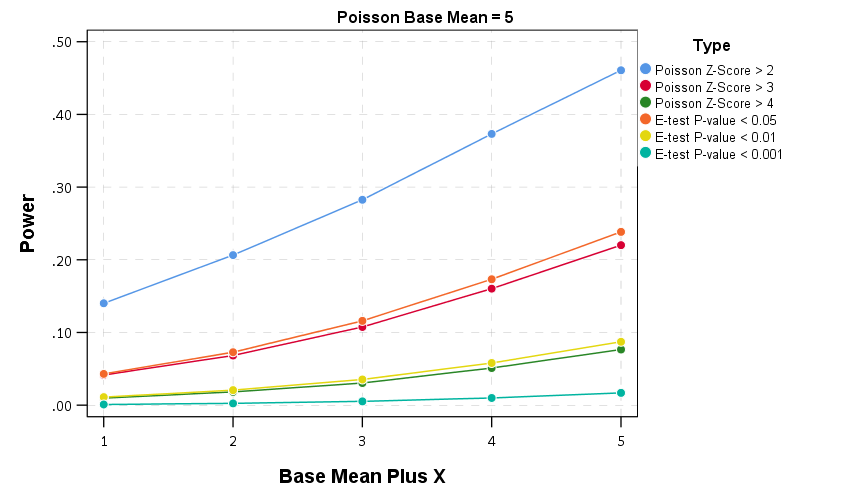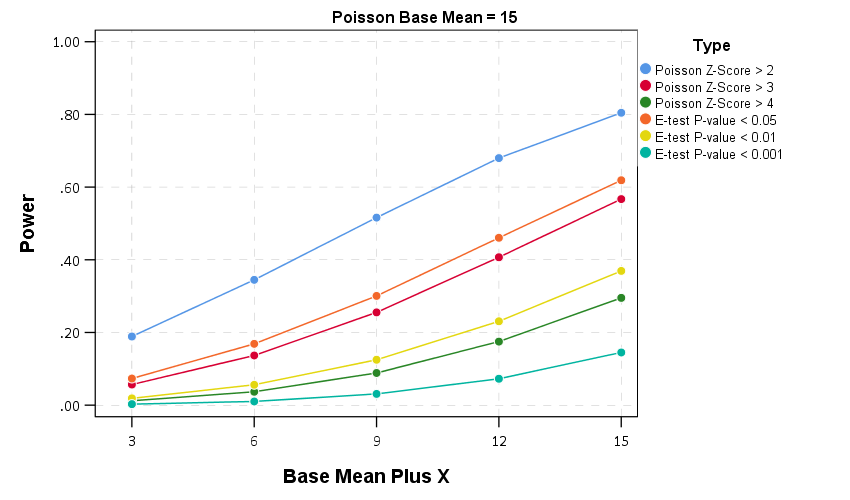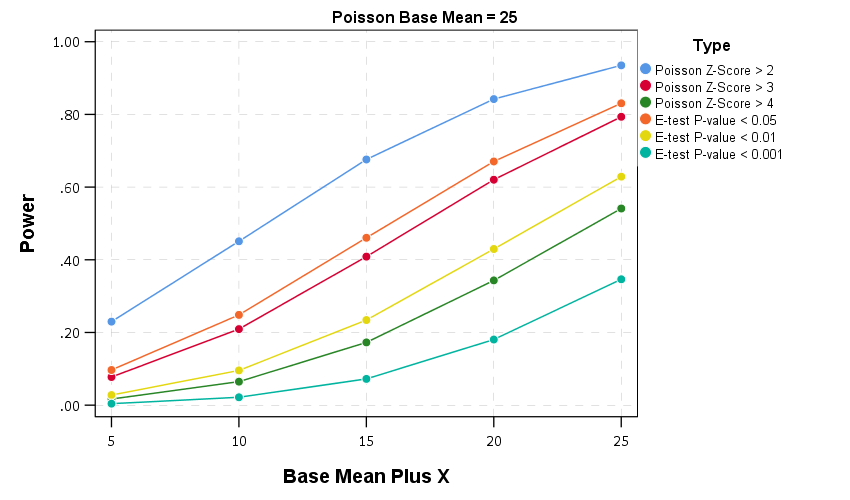So you can see there is a steep trade-off in power with setting a higher threshold for either the Poisson Z score or the E-test. The curves for the Z score of above 3 and above 4 basically follow the E-test curves for <0.05 and <0.01. The Poisson Z-score of over 2 has a much higher power, but of course that comes with the much higher false positive rate as well.

For the lowest base mean of 5, even doubling the underlying rate to 10 still has quite low power to uncover the difference via any of these tests. With bases of 15 and 25 doubling gets into a bit better range of at least 0.5 power or better. Despite the low power though, the way these statistics are typically implemented in crime analysis departments along regular intervals, I think doing a Poisson Z-score of > 3 should be the lowest evidentiary threshold an analyst should use to say "lets look into this increase further".

Of course since the E-test is better behaved than my Poisson Z-score you could swap that out as well. It is a bit harder to implement as a simple spreadsheet formula, but for those who do not use R or Python I have provided an excel spreadsheet to test the differences in two simple pre-post counts in the data files to replicate this analysis.

# In conclusion

I see a few things to improve upon this work in the future.

First is that given the low power, I wonder if there is a better way to identify changes when monitoring many series but still be able to control the false positive rate. Perhaps some lower threshold for the E-test but simultaneously doing a false discovery rate correction to the p-values, or maybe some way to conduct partial pooling of the series into a multi-level model with shrinkage and actual parameters of the increase over time.

A second is a change in the overall approach about how such series are monitored, in particular using control charting approaches in place of just testing one vs another, but to identify consistent rises and falls. Control charting is tricky with crime data — there is no gold standard for when an alarm should be sounded, crime data show seasonality that needs to be adjusted, and it is unclear when to reset the CUSUM chart — but I think those are not unsolvable problems.

One final thing I need to address with future work is the fact that crime data is often over-dispersed. For my Poisson Z-score just setting the threshold higher with data seemed to work ok for real and simulated data distributed like a negative binomial distribution, but I would need to check whether that is applicable to the e-test as well. I need to do more general analysis to see the typical amounts of over/under dispersion though in crime data to be able to generate a reasonable simulation though. I can probably use NIBRS data to figure that out — so for the next blog post!

1. Note the e-test is not defined when both values are zero.

2. You can technically calculate the exact power of the e-test, see the cited Ksrishnamoorthy & Thomson (2004) article that introduces it. For simplicity I am just doing the simulation for both my Poisson Z-scores and the e-test here.

# Understanding Uncertainty – crime counts and the Poisson distribution

A regular occurrence for me when I was a crime analyst went along the lines of, "There was a noteworthy crime event in the media, can you provide some related analysis". Most of the time this followed one or multiple noteworthy crimes that caught the public’s attention, which could range from a series of thefts from vehicles over a month, the same gas station being robbed on consecutive days, or a single murder.

Any single violent crime is awful, and this is not meant to deny that. But often single noteworthy events are often misconstrued as crime waves, or general notions that the neighborhood is in decline or the city is a more dangerous place now than it ever was. The media is intentionally hyperbole, so it is not an effective gauge whether or not crime is really increasing or decreasing. Here I will show an example of using the Poisson distribution to show whether or not a recent spree of crimes is more than you would expect by chance.

So lets say that over the course of 20 years, the mean number of homicides in a jurisdiction is 2. Lets also say that in the year so far, we have 5 homicides. Ignoring that the year has not concluded, what is the probability of observing 5 or more homicides? Assuming the number of homicides is a Poisson distributed random variable (often not too unreasonable for low counts over long time periods) the probability is 5.7%. Small, but not totally improbable. To calculate this probability it is just 1 minus the cumulative distribution function for a Poisson distribution with the given mean. This can be calculated easily in R by using `ppois`, i.e. `1 - ppois(5-1,2)` (just replace `5` with your observed count and `2` with your mean). Note that I subtract 1 from 5, otherwise it would be testing the probability of over 5 instead of 5 or more. For those analysts using Excel, the formula is `=1 - POISSON.DIST(5-1,2,TRUE)`. For SPSS it would be `COMPUTE Prob = 1 - CDF.POISSON(5-1,2)`.

The reason for making these calculations is specifically to understand chance variations given the numbers historically. For the crime analyst it is necessary to avoid chasing noise. For the public it is necessary to understand the context of the current events in light of historical data. For another example, say that the average number of robberies in a month is 15, what is the probability of observing 21 or more robberies? It is 8%, so basically you would expect this high of a number to happen at least one time every year (i.e. 0.08*12~1). Without any other information, there is little reason for a crime analyst to spend extra time examining 21 robberies in a month based on the total number of events alone. Ditto for the public there is little reason to be alarmed by that many robberies in a month given the historical data. (The analyst may want to examine the robberies for other reasons, but there is no reason to be fooled into thinking there is an unexpected increase.)

Here I’ve ignored some complications for the sake of simplicity in the analysis. One is that crime may not be Poisson distributed, but may be under or over-dispersed. In the case of over-dispersion (which seems to happen more often with crime data) the series likely has a higher number of 0’s and then high bursts of activity. In this case you would expect the higher bursts more often than you would with the Poisson distribution. For under-dispersed Poisson data, the variance is smaller than the mean, and so higher bursts of activity are less likely. These are fairly simple to check (at least to see if they are grossly violated), either see if the mean approximately equals the variance, or draw a histogram and superimpose a density estimate for the Poisson distribution. This also ignores seasonal fluctuations in crime (e.g. more burglaries occur in the summer than in the winter).

Even if you do not like making the Poisson assumption a very simple analysis to conduct is to plot the time series of the event over a long period. The rarer the crime the larger aggregation and time series you might need, but this is pretty straightforward to conduct with a SQL query and whatever program you use to conduct analysis. If it is for UCR crime counts, you can try going onto the UCR data tool to see if your jurisdiction has historical annual data going back to 1985. My experience is the vast majority of crime waves depicted in the media are simply chance fluctuations, clearly visible as such just by inspecting the time series plot. Similarly such a plot will show if there is an increase or a decrease compared to historical numbers. Another simple analysis is to take the current numbers and rank them against, say the prior 50 to 100 values in the series. If it is abnormal it should be the the highest or very near the highest in those prior values.

Another complication I have ignored is that of multiple testing. When one is constantly observing a series, even a rare event is likely to happen over a long period of observation. So lets say that in your jurisdiction on average there are 3 domestic assaults in a week, and one week you observe 9. The probability of observing 9 or more is 0.003, but over a whole year the probability of this happening at least once is around 18% (i.e. `1 - ppois(8,3)^52` in R code). Over the course of 10 years (around 520 weeks) we would expect around 2 weeks to have 9 or more domestic assaults (i.e. `520*(1-ppois(8,3))`). (This probability goes up higher if we consider sliding windows, e.g. 9 or more domestic assaults in any 7 day span, instead of just over different weeks.) These statistics make the assumption that events are independent (likely not true in practice) but I rather make that false assumption to get a sense of the probability then rely on gut feelings or opinions based on the notoriety of the recent crime(s).

The title for this blog post is taken from David Spiegelhalter’s site Understanding Uncertainty, and that link provides a synonymous example with the recent cluster of plane crashes.

# Poisson regression and crazy predictions

Here is a problem I’ve encountered a few times in my own work (and others) with Poisson regression models and the exponential link function. It came up recently in some discussions on the scatterplot blog by Jeremy Freese (see 1 & 2) critiquing the PNAS paper on the effect of female named hurricanes on death tolls, so I figured I would expand up those thoughts a little here.

So the problem is when you estimate a Poisson regression model is that the exponential link function can become explosive for explanatory variables that have a large range. So to be clear, we have a Poisson regression model of the form (here E[Y] mean the expected value of Y):

``````log(E[Y]) = B1*(X)
E[Y]  = e^(B1*X)``````

If X has a small range this may be fine, but if X has a large range it can become problematic. Consider if Y are hurricane deaths, X is monetary damage of the hurricane, and B1 = 0.01. Lets say the monetary damage ranges from 1 to 1000 (imagine these are in thousands of dollars, so range between \$1,000 and \$1 million). What happens with the predictions?

``````E[Deaths] = e^(0.01*   1) =     1.01
E[Deaths] = e^(0.01*   5) =     1.05
E[Deaths] = e^(0.01*  10) =     1.11
E[Deaths] = e^(0.01*  50) =     1.65
E[Deaths] = e^(0.01* 100) =     2.71
E[Deaths] = e^(0.01* 500) =   148
E[Deaths] = e^(0.01*1000) = 22026``````

These predictions are invariant to linear transformations – that is Z-scoring X in the original units doesn’t change the predictions (the same as expressing X in [dollars/1000] doesn’t make any difference than just by including [X] on the right hand side). The linear predictor of B1 will simply be scaled by the appropriate inverse transformation. Also I’d note that expressed in terms of incident rate ratios the effect would be e^0.01=1.01. This appears on its face a totally innocuous effect, and only in consideration of the variation in X does it appear to be absurd.

You can see that if the range of X were smaller, say between 1 and 100, the predictions might be fine. The predictions between 1 and 100 only vary by 1.7 deaths. The problem with these explosive predictions at larger values is that they are nonsense for most of social scientific research. A simple sanity check to see if this is occurring is to check the predicted value from your Poisson regression equation at the low end of X versus the high end (and just pretend all of the other explanatory variables are set to 0) exactly as I have done here. If the high end is crazy, you will need to consider some alternative model specification (or be very clear that the model can not be extrapolated to the larger values of X).

A useful alternate parametrization is simply to log X, and in this case when exponentiating the right hand side, it will make the predictor a power of the original metric.

So imagine we fit the model:

``````log(E[Y]) = B2*(log(X))
E[Y]) = e^(B2*log(X))
= x^B2``````

Lets say here that B2 = 0.5. What happens to our predictions again?

``````E[Deaths] =    1^0.5 =  1
E[Deaths] =    5^0.5 =  2.2
E[Deaths] =   10^0.5 =  3.2
E[Deaths] =   50^0.5 =  7.1
E[Deaths] =  100^0.5 = 10
E[Deaths] =  500^0.5 = 22
E[Deaths] = 1000^0.5 = 32``````

Those predictions look a little bit easier to swallow at the larger ranges. Notice also the differences in predictions in the smaller stages? There is more discrimination for the smaller values than on the original scale, but the larger values are suppressed. Lets consider the predictions side by side for easier comparison.

``````   X      B1   B2
-      --   --
1       1    1
5       1    2
10       1    3
50       2    7
100       3   10
500     148   22
1000   22026   32``````

A frequent problem with logging the explanatory variables is that they contain zeroes. A simple alternative is to treat log(0) as 0 and then have a separate dummy variable equal to 1 when X = 0. This model may not make Occam happy, as it implies a discontinuity at 0, but it is in my opinion a small price to pay. Also if there are a lot of zeroes this doesn’t strike me as totally unrealistic to have a mixture of what happens at 0 and then what happens at the higher values. So the full model written out would be:

``log(E[Y]) = B3*D + B4*(log(X))``

But the model is essentially discontinuous. When X=0, we treat log(X)=0 and D=1, so the model reduces to;

``log(E[Y]) = B3*D :When X = 0``

When X>0, D=0 and the model reduces to:

``log(E[Y]) = B4*(log(X)) :When X > 0``

Now, it certainly would be weird if B3>>0, as this would imply a high spike at 0, and then at the X value of 1 Y goes back down to 1 and then increases with X. If we expect B4 to be positive, then a negative value of B3 (or very close to 0) would make the most sense. It is still a discontinuity in the function, but one that may make theoretical sense. So imagine we fit the equation `log(E[Y]) = B3*D + B4*(log(X))`, lets say B4 is 0.5 (the same as B2), and that B3 is equal to -0.1. This would then make the set of predictions go:

``````E[Deaths] =  e^-0.01 =  0.9
E[Deaths] =    1^0.5 =  1.0
E[Deaths] =    5^0.5 =  2.2
E[Deaths] =   10^0.5 =  3.2
E[Deaths] =   50^0.5 =  7.1
E[Deaths] =  100^0.5 = 10
E[Deaths] =  500^0.5 = 22
E[Deaths] = 1000^0.5 = 32``````

So in this made up example the discontinuity pretty much fits right in with the rest of the function. We may consider other non-linear transformations of X as well (splines or higher powers) but frequently an additional problem is that the bulk of the data lie in the lower end of the range. So for our dollars if it was highly right skewed, there may only be a few values at 100 or higher. These can be highly influential if you use powers of X (e.g. include X^2, X^3 etc. on the right hand side) – so splines are a better choice – but essentially no matter how you fit the function it will be hard to verify the fit at these values or extrapolate to those tails. So the fit in the original function may be fine – but it just implies unrealistic marginal effects in the tails.

So how do we verify one equation over the other? Visualizing count data in scatterplots tend to be harder than visualizing continuous data, especially if there is a stock pile of data at 0. The problem is simply exacerbated if the explanatory variable has a similar right skew, there will be a large mass near the origin of the plot and very sparse everywhere else.

My simple suggesting is to just bin the data at X values, which is very simple if X is integer valued, and then plot the mean and standard error of the Y value within those bins. As Poisson regression and its variants rely on asymptotic properties, if the error bars are too variable to deduce a pattern you should be concerned your sample size isn’t large enough to begin with.

If the bulk of the data only have a small range over X, then it will be hard in practice to differentiate between the two model parametrizations I suggest here (with the typical noisy data we have in the social sciences). So you may prefer logging the X variable simply to prevent the dramatic explosions in the tails of the data right from the start.

I do feel comfortable saying that if the ratio of the smallest to largest value for the independent variable is over 100, you should check the predictions of the exponential link function very closely (if the smallest value is 0 just estimate the ratio as if the smallest value is 1). If this ratio is 100 or larger, unless the linear predictor in the Poisson regression equation is very small (<<.01) the predictions may explode into very implausible ranges for the larger X values.